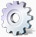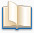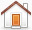How To Calculate Interest Rate Differential

Interest Rate Differential (IRD) Calculation:

PENALTY = MORTGAGE BALANCE x DIFFERENTIAL x MONTHS REMAINING / 12 MONTHS

Example:

1. \$100,000 mortgage at 9% interest rate with 24 months remaining.
2. Lenders current 2-year interest rate is 6.5%.
3. Differential is 2.5% (9%-6.5%).

IRD calculation: \$100,000 * 2.5% * 24 months / 12 months = \$5,000.

When calculating the IRD differential the lender will use the posted interest rate at the time you obtain your mortgage and not the current posted rate. Most consumers obtain their mortgages at discounted rates and do not readily know the posted rate of the lender at the time they obtained their mortgage.

This IRD calculation is only to be used as a guide to understand the calculation. Contact your lender for the exact payout amount.ToolsGuides